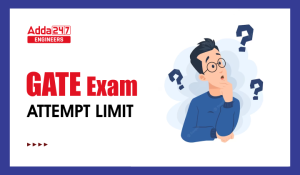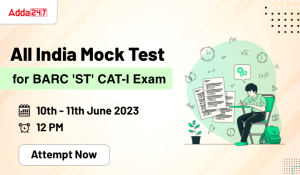Engineering Jobs   »   QUIZ: CIVIL (03-06-2020)

# QUIZ: CIVIL (03-06-2020)

Quiz: Civil Engineering
Exam: UKSSSC-JE
Topic: RAILWAY ENGINEERING

Each question carries 1 mark
Negative marking: 1/4 mark
Time: 10 Minutes

Q1. The sharpness of the curve is designated by its _____________.
(a) Normal chord
(b) Intersection angle
(c) Degree of curvature
(d) Tangent distance

Q2. Coning of wheels is provided
(a) to check lateral movement of wheels
(b) to avoid damage to the inner faces of rails
(c) to avoid discomfort to passengers
(d) all of these

Q3. If L is the length of a rail and R is the radius of a curve, the versine h for the curve is …………
(a) a=L/4R
(b) a=L²/4R
(c) h=L²/8R
(d) h=L²/16R

Q4. Maximum degree of curvature for B.G. track is: –
(a) 20°
(b) 10°
(c) 16°
(d) 15°

Q5. The shift of the transition curve of radius 300m and length 48m is
(a) 0.32 m
(b) 0.42 m
(c) 0.52 m
(d) 0.62 m

Q6. What is the curve resistance for a 50 tonnes train on a BG track on a 4° curve?
(a) 0.05 tonne
(b) 0.06 tonne
(c) 0.08 tonne
(d) 0.01 tonne

Q7. In India, the ruling gradient provided in plains for one locomotive train is
(a) 1 IN 150 to 1 IN 200
(b) 1 IN 200 to 1 IN 250
(c) 1 IN 250to 1 IN 300
(d) 1 IN 300 to 1 IN 350

Q8. The total angle of deflection of a transition curve is equal to: –
(a) Spiral angle
(b) Spiral angle/2
(c) Spiral angle/3
(d) Spiral angle/4

Q9. The type of transition curve used in Indian railways, is
(a) spiral
(b) cubic parabola
(c) lemniscate
(d) S-curve

Q10. Following method is used to calculate the crossing angle
(a) right angle method
(b) centre line method
(c) isosceles triangle method
(d) all of these

SOLUTION

S1. Ans.(c)
Sol. The sharpens of the curve is designated by its degree of curvature.

S2. Ans.(d)
Sol. Coning of wheel is provide
(i) To check lateral movement of wheels
(ii) To reduce wear & Tear of wheels
(iii) To avoid discomfort of passengers
(iv) To avoid damages to the inner faces of rails.

S3. Ans.(c)
Sol. ▭(Versine of curve (h)= L^2/8R )
L = Length of rail

S4. Ans.(b)
Sol.
Gauge Maximum degree of curve
2. Meter Gauge (MG)
3. Narrow Gauge (NG) 10°
16°
40°

S5. Ans.(a)
Sol. Given, R = 300m.
L = 48 m.
Shift (S) = ?
S = L^2/(24 R)
=((48)²)/(24×300)
= 0.32 m.

S6. Ans.(c)
Sol. Given, Degree (D°) = 4°
Total weight of Train (W) = 50 Tonnes
The curvature resistance for BG = 0.0004 WD°
= 0.0004 × 50 × 4
= 0.08 Torne

S7. Ans.(a)
Sol. The ruling gradient is provided for one locomotive train is a following –
Plain Areas → 1 in 150 to 1 in 200
Hilly Areas → 1 in 100 to 1 in 150

S8. Ans.(c)
Sol. ▭(Deflection anlgle (δ)=(spiral angle (α))/3)

S9. Ans.(b)
Sol. The type of transition curve used in India railways, it cubic parabola.

S10. Ans.(d)
Sol. There are following method used to calculate crossing angle-
(i) Right angle triangle method.
(ii) Centre line method.
(iii) Isocele triangle method

Sharing is caring!

•GATE Result 2023 Out, Download Result, C...
•GATE 2023 Admit Card Out, Download Link ...
•JSSC JE Recruitment 2023 Out, Apply Onli...
•GATE Exam Attempt Limit, Check Here
•How to Prepare for GATE 2024 in 6 Months
•All India Mock Test For DDA JE Exam 2023...
•All India Mock Test for BARC 'ST' CAT-I ...
•DDA JE Recruitment 2023 Out, Apply Onlin...